# Operators in C Programming

## 12 Jan Operators in C Programming

Operators perform operations by taking one or more value, to give another value. These operations are performed on variables and values. In this lesson, we will learn about operators in C Programming.

For example:  a + b

The types of operators is explained here with an example, where a = 5, b =10. Here are the operators in C Programming:

## C Arithmetic operators

Arithmetic operators perform arithmetical operations, such as addition, subtraction, division, multiplication etc.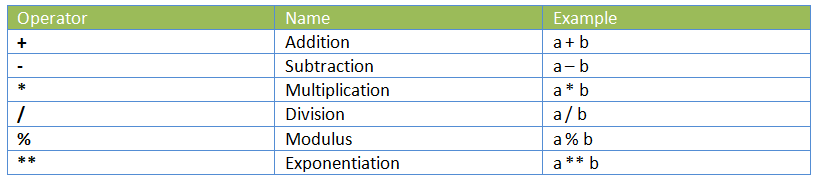The following example shows how to work with Arithmetic operators in C Programming,

Here’s the output,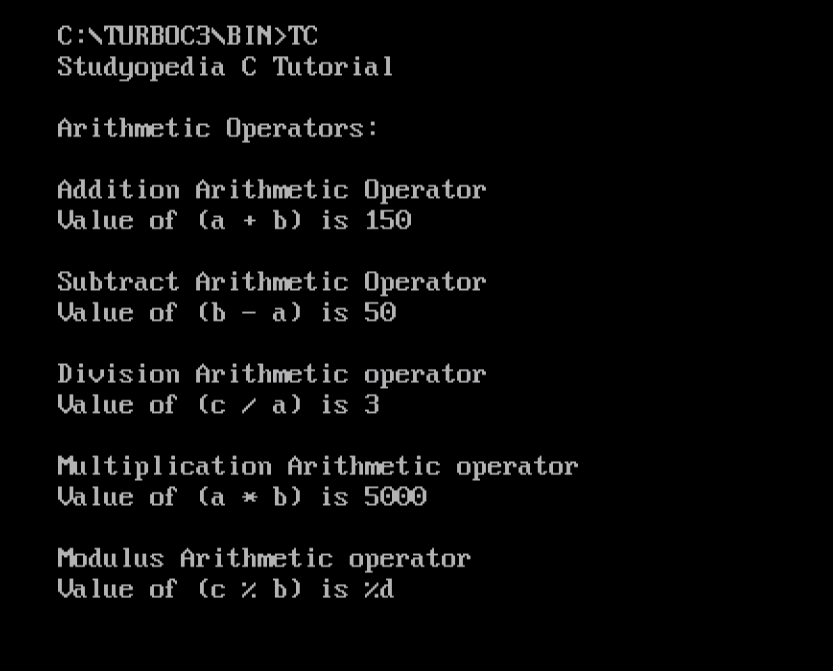## C Assignment Operator

Use the Assignment Operator when you need to assign a value to a variable. Assignment means assigning the value of right operand(s) to the left operand.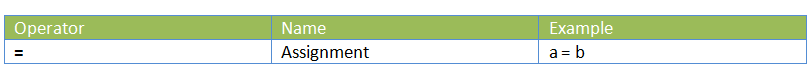## C Arithmetic Assignment Operator

Use the Arithmetic Assignment Operator when you need to perform arithmetic operations such as add, subtract, divide, multiple, etc and at the same time assign a value .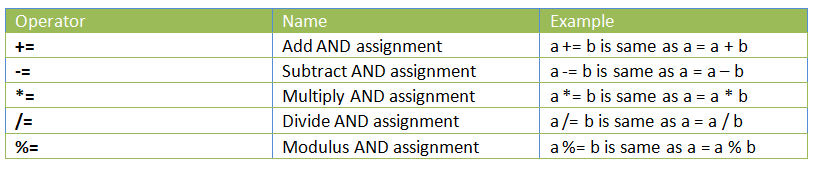The following example shows how to work with Arithmetic Assignment Operators in C Programming,

Here’s the output,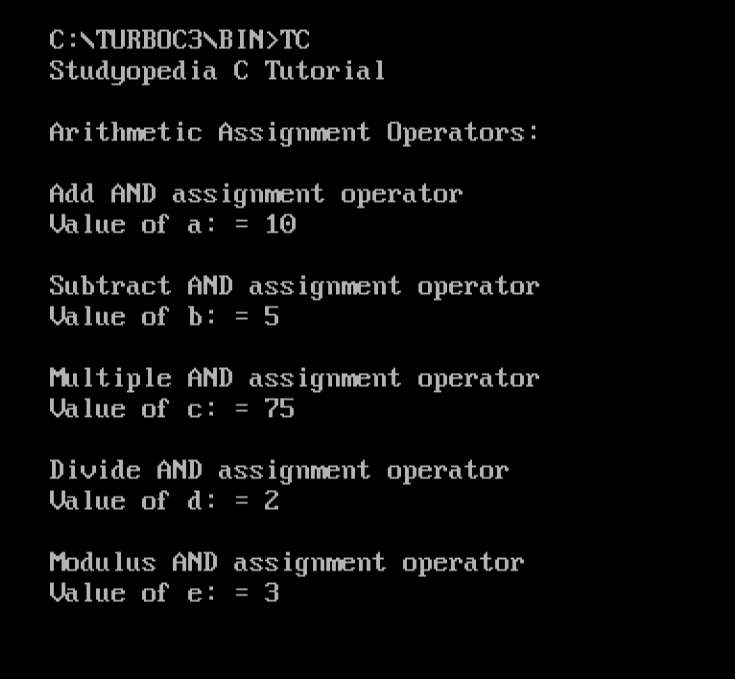## C Relational/ Comparison operators

Compare two values with relational operators, which is also known as Comparison Operators.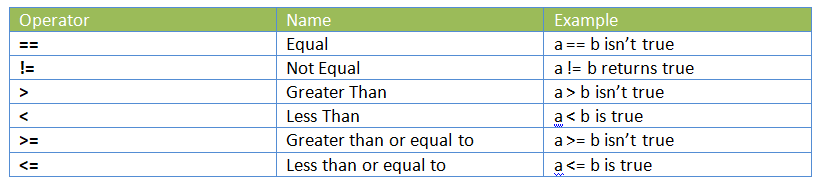The following example shows how to work with Relational Operator in C Programming,

Here’s the output,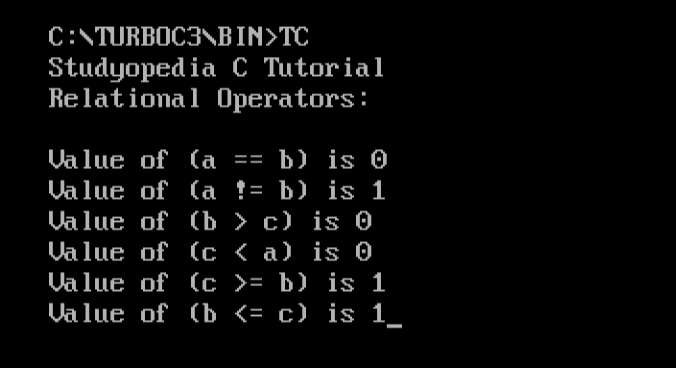## C Logical Operators

Logical operators combine conditional statements. For an example, we’re considering Boolean variables a and b,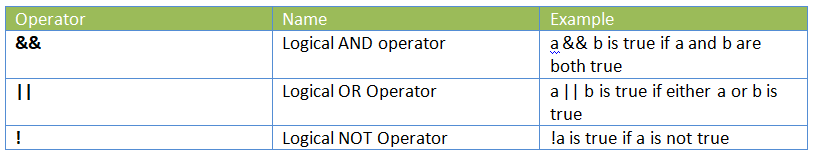The following example shows how to work with Logical Operator in C Programming,

Here’s the output,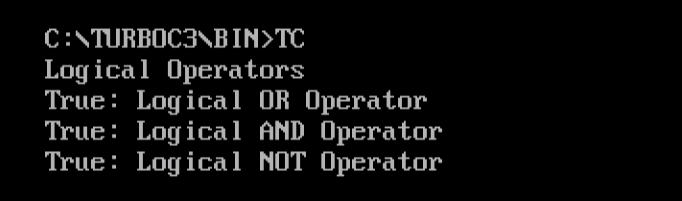## C Unary operators

Unary operators include pre as well as post increment and decrement operators,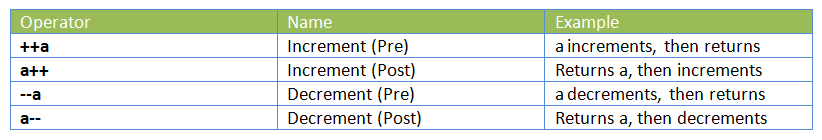The following example shows how to work with Unary Operators in C Programming,

Here’s the output,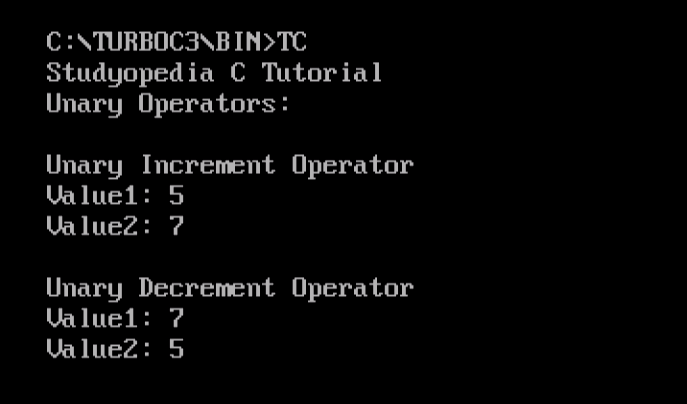## C  Conditional Operators

Conditional operator evaluates Boolean expressions, with has three operands. It is also known as the ternary operator. The role is to assign a value to a variable, from two given set options.

Here’s how to use it,

The following example shows how to work with Conditional Operator in C Programming,

Here’s the output,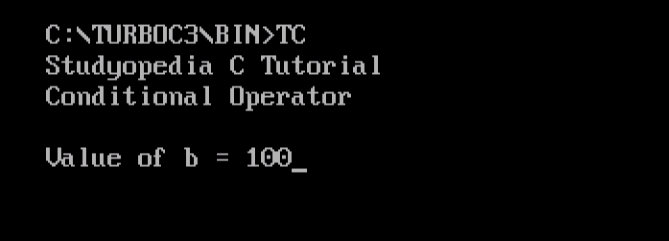In this lesson we learned about Operators in C Programming. We saw how to work with Arithmetic, Unary, Relational, Arithmetic Assignment and other operators.[email protected]

We work to create free tutorials for all.